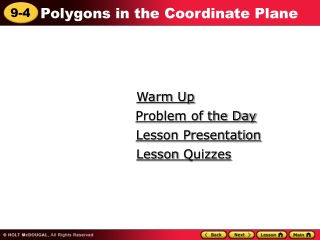DownloadDownload PresentationWarm Up

# Warm Up

Télécharger la présentation## Warm Up

- - - - - - - - - - - - - - - - - - - - - - - - - - - E N D - - - - - - - - - - - - - - - - - - - - - - - - - - -
##### Presentation Transcript

1. Warm Up Problem of the Day Lesson Presentation Lesson Quizzes

3. Problem of the Day Each side of a rectangle is doubled. How does the perimeter change? The perimeter is doubled.

4. Learn to draw polygons in the coordinate plane and find the lengths of their sides.

5. Helpful Hint Remember that in a rectangle, opposite sides have equal length.

6. Additional Example 1: Drawing Polygons in the Coordinate Plane Graph the triangle with vertices F(2, 5), G(4, 1), and H(0, 22). Graph each point on the coordinate plane. Then connect the points with straight lines to form a polygon.

7. Check It Out: Example 1 Graph the triangle with vertices A(1, 2), B(4, 2), and C(3, 1). Graph each point on the coordinate plane. Then connect the points with straight lines to form a polygon.

8. Additional Example 2: Finding Distance in the Coordinate Plane Find the perimeter of the rectangle. Step 1: Find the length and width. To find the length of the rectangle, count from left to right along the x-axis from –1 to 3. The length is 4 units long. To find the width, count up the y-axis from –2 to 4. The width is 6 units long.

9. Additional Example 2: Continued Step 2: Find the perimeter. Use the formula for the perimeter of a rectangle. Length of the rectangle = l Width of the rectangle = w Perimeter = 2l + 2w P = 2(4) + 2(6) = 20 The perimeter of the rectangle is 20 units.

10. Check It Out: Example 2 Find the perimeter of the rectangle. Step 1: Find the length and width. To find the length of the rectangle, count from left to right along the x-axis from 5 to 8. The length is 3 units long. To find the width, count up the y-axis from 0 to 10. The width is 10 units long.

11. Continued: Check It Out: Example 2 Step 2: Find the perimeter. Use the formula for the perimeter of a rectangle. Length of the rectangle = l Width of the rectangle = w Perimeter = 2l + 2w P = 2(3) + 2(10) = 26 The perimeter of the rectangle is 26 units.

12. Additional Example 3: Social Studies Application Candice’s house is at the corner of 3rd Avenue and 7th Street. She walks from her house west to Minus 2nd Avenue, then south to 4th Street, then east to 3rd Avenue, and then back home. How far did she walk? Step 1: Plot the points and draw the rectangle. Step 2: Find the length and width of the rectangle. Step 3: Find the perimeter. Perimeter = 2l + 2w P = 2(5) + 2(3) = 16 Candice walked 16 blocks.

13. Check It Out: Example 3 A polygon has vertices at (2, 4), (3, 4), (3, 1), and (2, 1). What type of polygon is this? What is its perimeter? The polygon is a rectangle. The perimeter is 8 units

14. Lesson Quizzes Standard Lesson Quiz Lesson Quiz for Student Response Systems

15. 1 1 2 2 Lesson Quiz Part I 1. Graph the rectangle with vertices (2, 2), (2, 5 ), (-3, 5 ), and (-3, 2).

16. Lesson Quiz Part II 2. Find the perimeter of the rectangle. 18 units

17. Lesson Quiz Part III 3. Albert set up his vegetable garden using a coordinate system with units of feet. The corners of the garden are at (4, -2), (4, 3), (-1 ,3), and (-1, -2). What is the perimeter of the garden? 20 feet

18. Lesson Quiz for Student Response Systems 1. Find the perimeter of the rectangle. A. 12 units B. 24 units C. 26 units D. 42 units

19. Lesson Quiz for Student Response Systems 2. A polygon has vertices at (2, 4), (3, 4), (3, 8), and (2, 8). What is its perimeter? A. 2 units B. 8 units C. 10 units D. 18 units